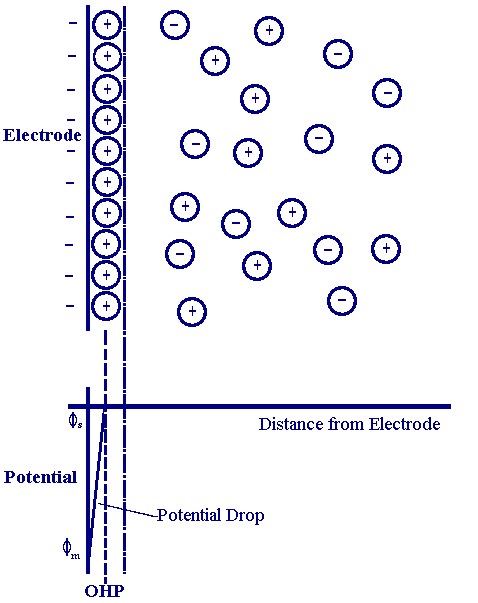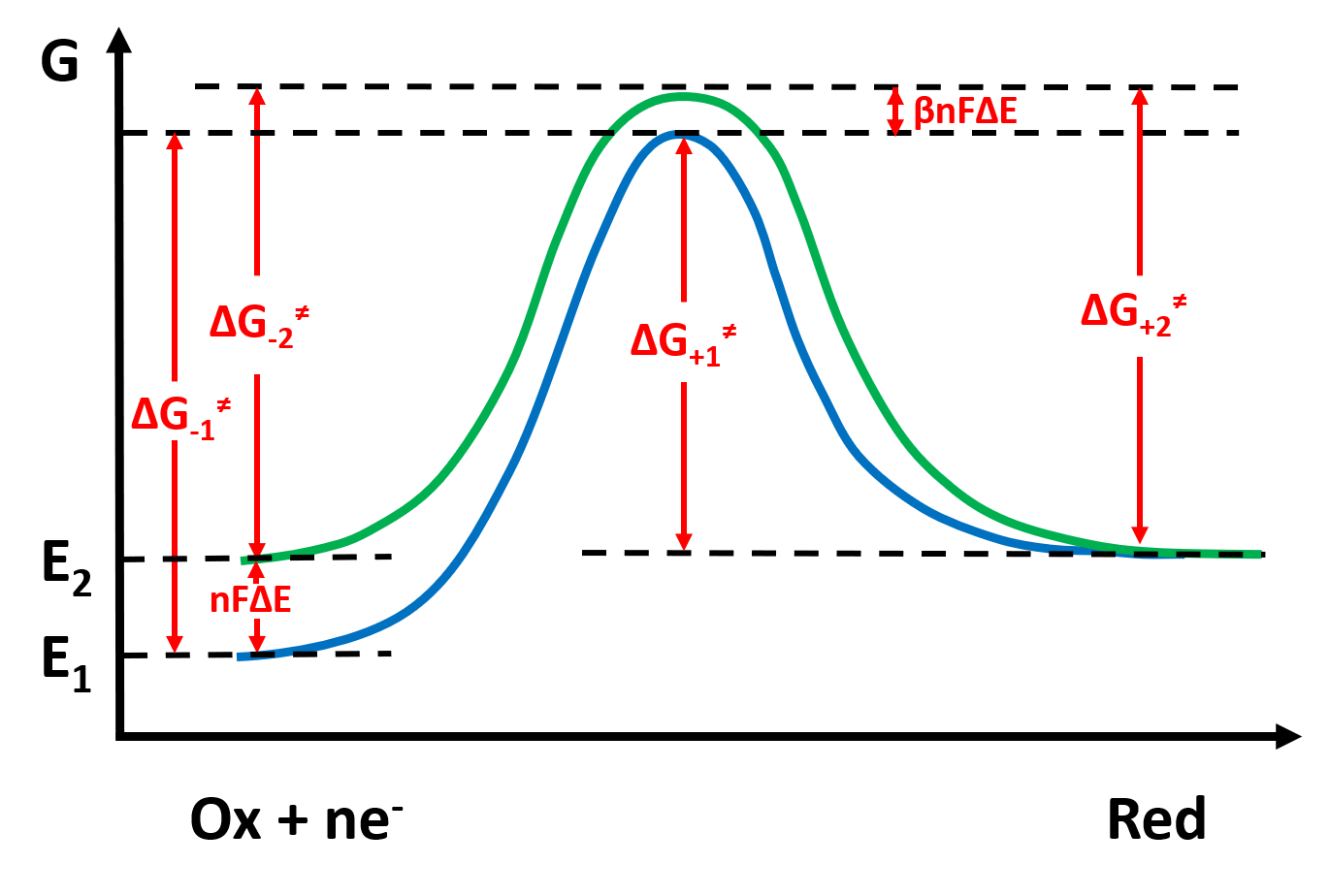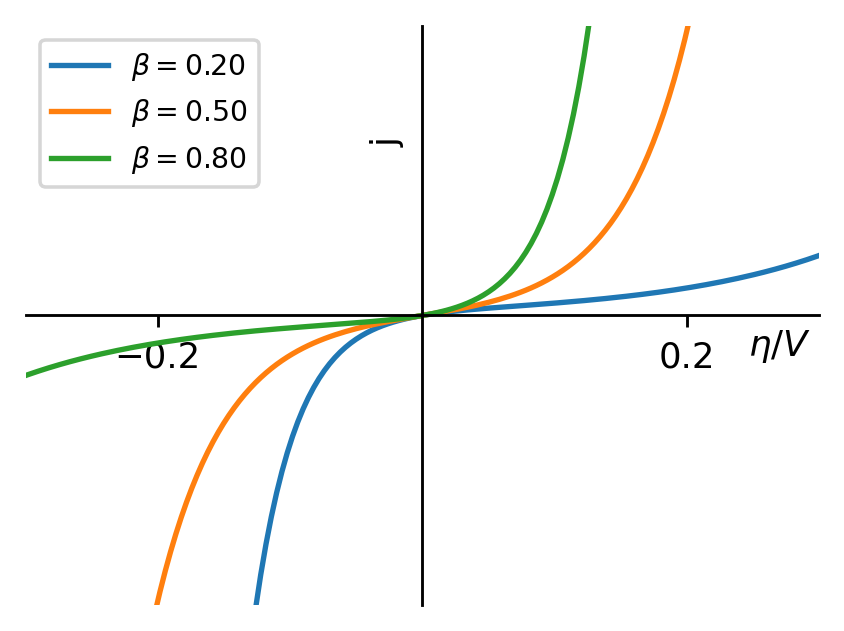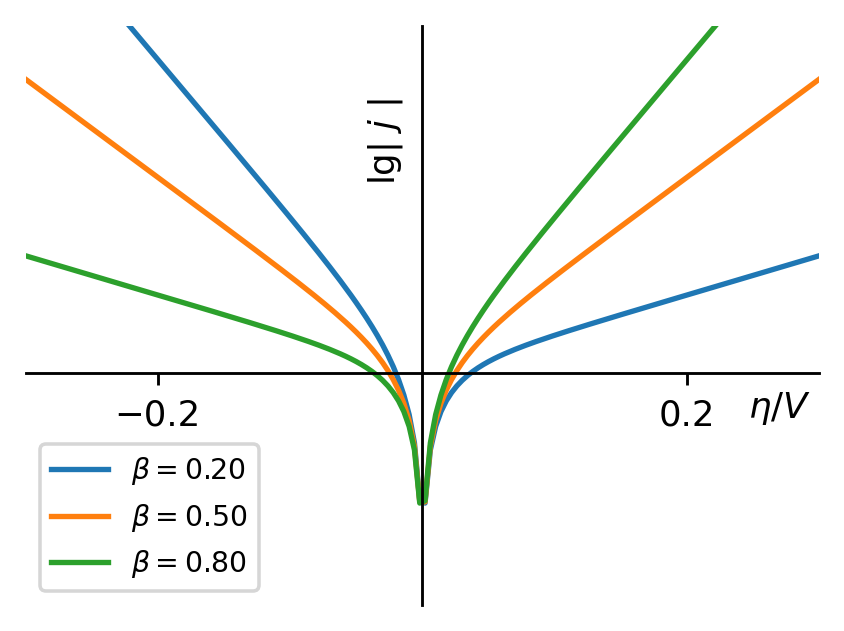## 1. 过电势的产生

• 浓差极化是由于电极表面反应物的浓度与本体溶液间存在差别导致电势值发生偏离，是传质扩散过程的影响。极谱分析法就是依据扩散速度与浓度的关系测量离子浓度的。
• 电化学极化则源于电极的电荷转移和氧化还原过程，反应需克服一定的势垒导致需要额外的能量才能使反应正向进行。
• 电阻极化是由于电极表面生成了导电性很差的物质。

## 2. 模型的建立

$Ox\ +\ ne^- \ \rightleftharpoons \ Red$• 理论基础：过渡态理论（刻画反应过程，计算反应速率）
• 电化学模型：双电层模型（描述电势的变化）
• 假设：电化学极化（过电势仅由电化学极化引起）、电解质溶液浓度大（使得电势的变化近似只发生于双电层附近）

## 3. Nernst方程与B-V方程的推导

\begin{aligned} v_-(E_1)&=k_-\cdot c_{Ox}\cdot \exp\left({-\frac{\Delta G_{-}^{\neq}(E_1)}{RT}}\right)\\ j_-(E_1)&=-nFc_{Ox}k_-\exp\left({-\frac{\Delta G_{-}^{\neq}(E_1)}{RT}}\right) \end{aligned}

\begin{aligned} v_-(E_2)&=k_-\cdot c_{Ox}\cdot \exp\left({-\frac{\Delta G_{-}^{\neq}(E_1)+(1-\beta)nF\Delta E}{RT}}\right)\\ j_-(E_2)&=-nFc_{Ox}k_-\exp\left({-\frac{\Delta G_{-}^{\neq}(E_1)+(1-\beta)nF\Delta E}{RT}}\right) \end{aligned}

$v_+(E_1)=k_+\cdot c_{Red}\cdot \exp\left({-\frac{\Delta G_{+}^{\neq}(E_1)}{RT}}\right)\\ j_+(E_1)=nFc_{Red}k_+\exp\left({-\frac{\Delta G_{+}^{\neq}(E_1)}{RT}}\right)\\ v_+(E_2)=k_+\cdot c_{Red}\cdot \exp\left({-\frac{\Delta G_{+}^{\neq}(E_1)-\beta nF\Delta E}{RT}}\right)\\ j_+(E_2)=nFc_{Red}k_+\exp\left({-\frac{\Delta G_{+}^{\neq}(E_1)-\beta nF\Delta E}{RT}}\right)$

\begin{aligned} j_-(E)&=-nFc_{Ox}k_-\exp\left({-\frac{(1-\beta)nFE}{RT}}\right)\\ j_+(E)&=nFc_{Red}k_+\exp\left({\frac{\beta nFE}{RT}}\right) \end{aligned}

\begin{aligned}j_-(E_r)&={\color{red}-}j_+(E_r)\\exp\left(\frac{nFE_r}{RT}\right)&=\frac{c_{Ox}}{c_{Red}}\cdot \frac{k_-}{k_+}\\\frac{nFE_r}{RT}&=\ln\left(\frac{c_{Ox}}{c_{Red}}\cdot \frac{k_-}{k_+}\right)\\E_r&=\frac{RT}{nF}\left(\ln\frac{c_{Ox}}{c_{Red}}+\ln \frac{k_-}{k_+}\right)\\E_r&=E_r^0+\frac{RT}{nF}\ln \frac{c_{Ox}}{c_{Red}}\end{aligned}

\begin{aligned} j_-(E)&=-nFc_{Ox}k_-\exp\left({-\frac{(1-\beta)nFE_r}{RT}-\frac{(1-\beta)nF\eta}{RT}}\right)\\ j_+(E)&=nFc_{Red}k_+\exp\left({\frac{\beta nFE_r}{RT}+\frac{\beta nF\eta}{RT}}\right) \end{aligned}

\begin{aligned} j_-(E)&=-j_0\exp\left({-\frac{(1-\beta)nF\eta}{RT}}\right)\\ j_+(E)&=j_0\exp\left({\frac{\beta nF\eta}{RT}}\right)\\ \end{aligned}

\begin{aligned} j=j_+(E)+j_-(E)=j_0\left[\exp\left({\frac{\beta nF\eta}{RT}}\right)-\exp\left({\frac{-(1-\beta) nF\eta}{RT}}\right)\right] \end{aligned}

## 3. Tafel方程的推导、不对称因子和交换电流密度的概念$|\eta|\gg RT/nF$时，还原电流相对于氧化电流可以忽略（反之亦然），则Butler-Volmer方程可略为一项。以阴极反应为例， $\eta<0$，则

\begin{aligned} j\approx j_-(E)=-j_0\exp\left({\frac{-(1-\beta) nF\eta}{RT}}\right) \end{aligned}

$\lg|j|=\lg j_0+\frac{1}{2.303}\frac{(1-\beta) nF }{RT}|\eta|$

$|\eta|=\frac{2.303RT}{(1-\beta) nF}\lg |j|-\frac{2.303RT}{(1-\beta)nF}\lg j_0$

$|\eta|=a+b\lg |j|$

$\eta=-\frac{2.303RT}{(1-\beta) nF}\lg (-j)+\frac{2.303RT}{(1-\beta)nF}\lg j_0$

$\eta=\frac{2.303RT}{\beta nF}\lg j-\frac{2.303RT}{\beta nF}\lg j_0$$\eta < 10 mV$时，可近似运用 $e^x\approx 1+x$，代入至Butler-Volmer方程中，可得

$j=j_0\frac{nF\eta}{RT}$

$j_0$对Tafel曲线的影响体现在其改变了截距而不是斜率。$j_0$增大会导致阴极过电势的数值升高（绝对值降低）和阳极过电势的降低。

$b=\frac{2.303RT}{(1-\beta)nF}$

## 4. 模型的局限性